# Python数据分析教程（二）：Pandas

## Pandas介绍

### Pandas导入

• Pandas是Python第三方库，提供高性能易用数据类型和分析工具
• Pandas基于NumPy实现，常与NumPy和Matplotlib一同使用
• 两个数据类型：Series, DataFrame
``import pandas as pd``

### Pandas与numpy的比较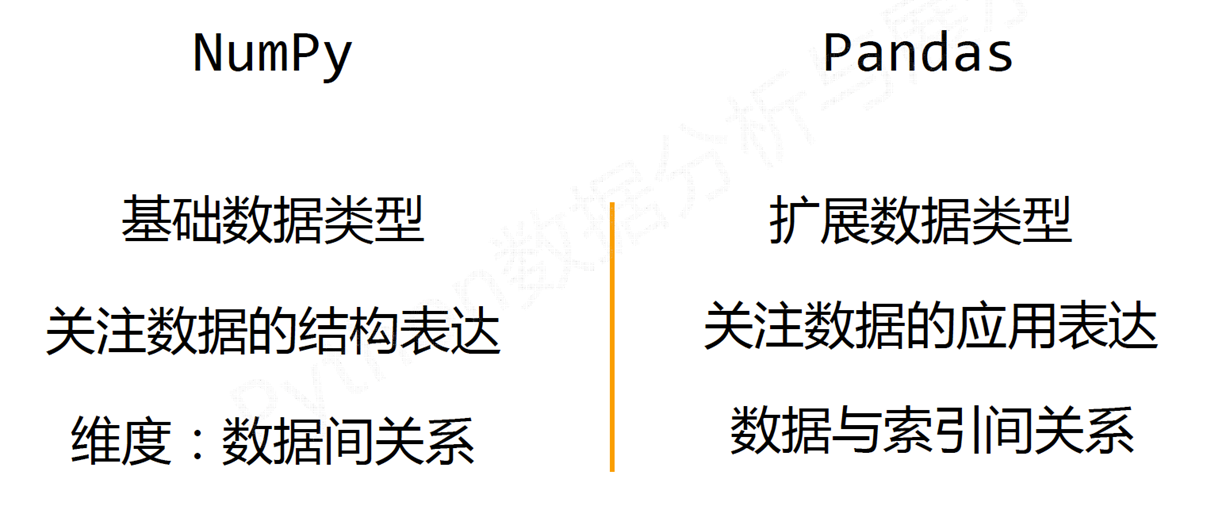## Pandas的Series类型### Pandas的Series类型的创建

Series类型可以由如下类型创建：

• Python列表，index与列表元素个数一致
• 标量值，index表达Series类型的尺寸
• Python字典，键值对中的“键”是索引，index从字典中进行选择操作
• ndarray，索引和数据都可以通过ndarray类型创建
• 其他函数，range()函数等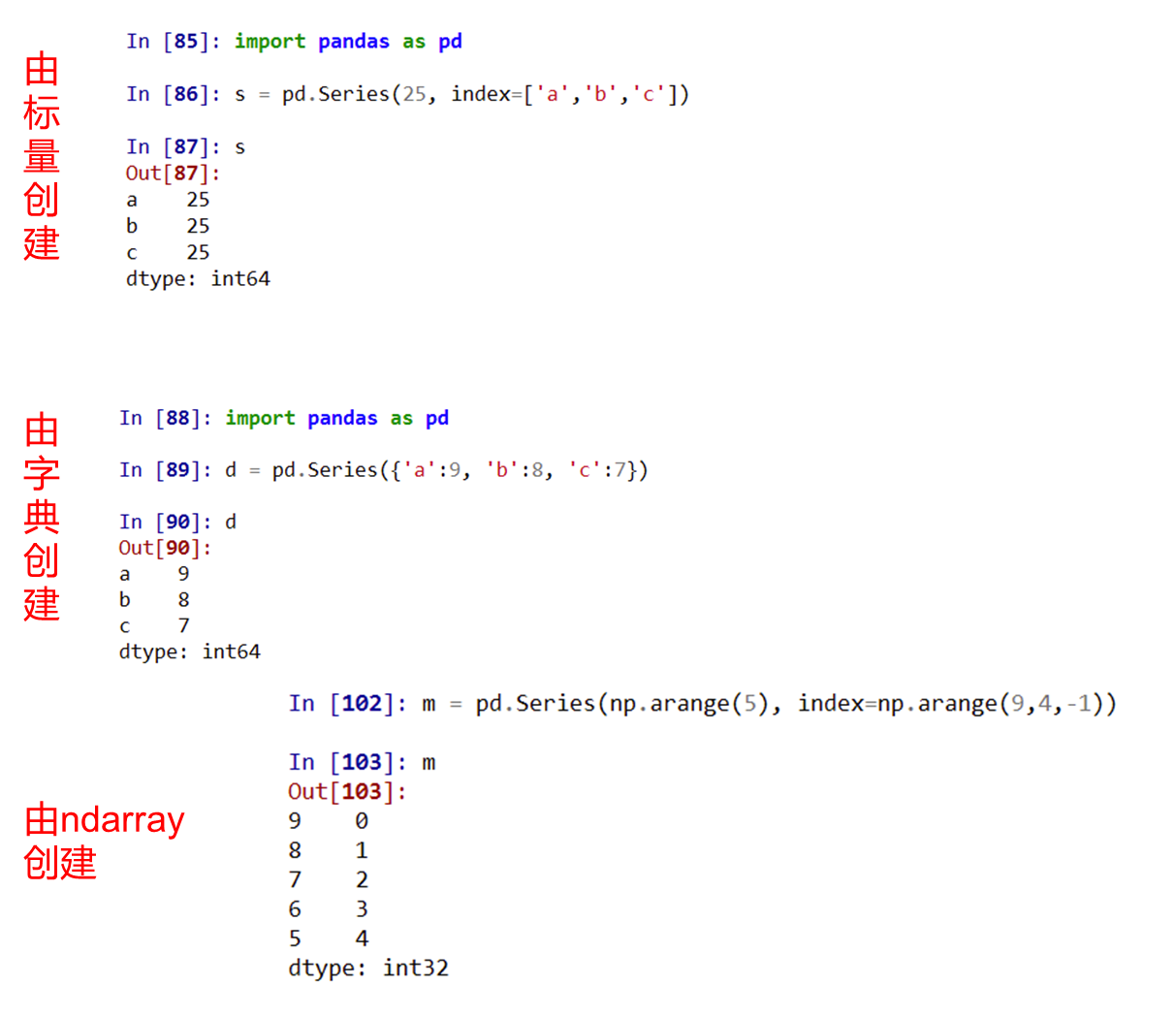### Pandas的Series类型的基本操作

Series类型包含index和values两个部分：

• index 获得索引
• values 获得数据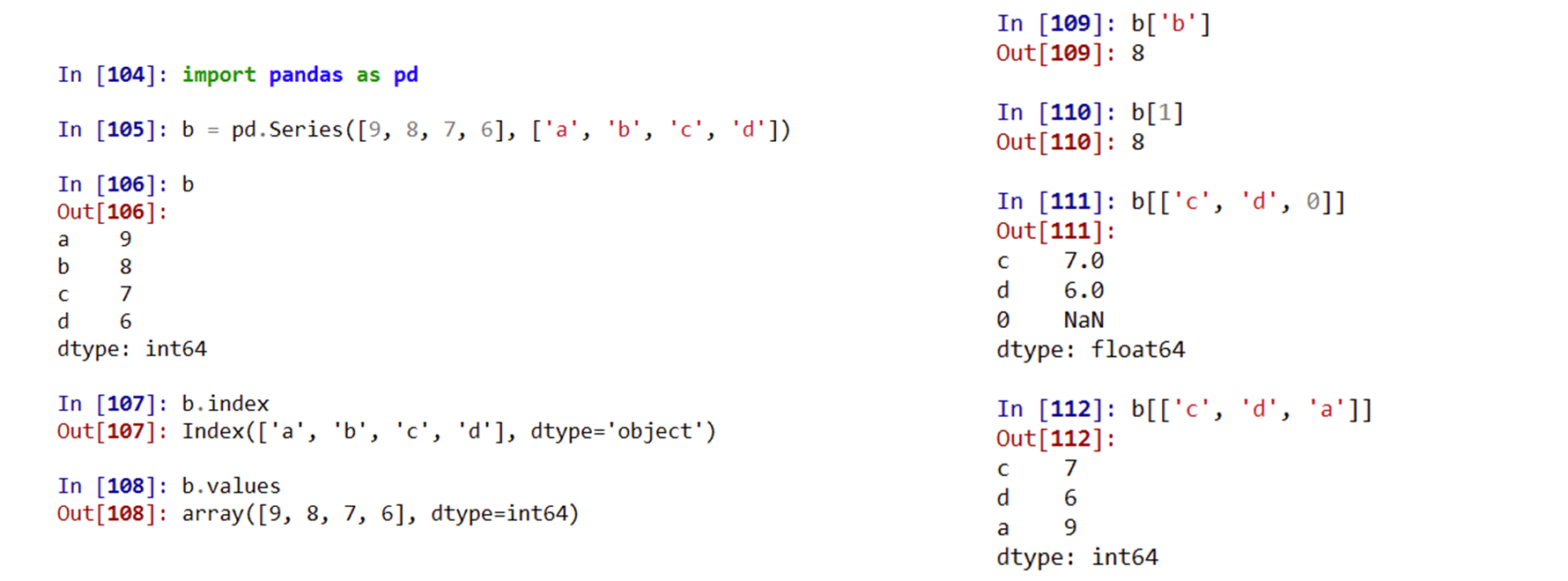## pandas的DataFrame类型

• DataFrame类型由共用相同索引的一组列组成

• DataFrame是一个表格型的数据类型，每列值类型可以不同

• DataFrame既有行索引、也有列索引

• DataFrame常用于表达二维数据，但可以表达多维数据

• DataFrame是二维带“标签”数组

• DataFrame基本操作类似Series，依据行列索引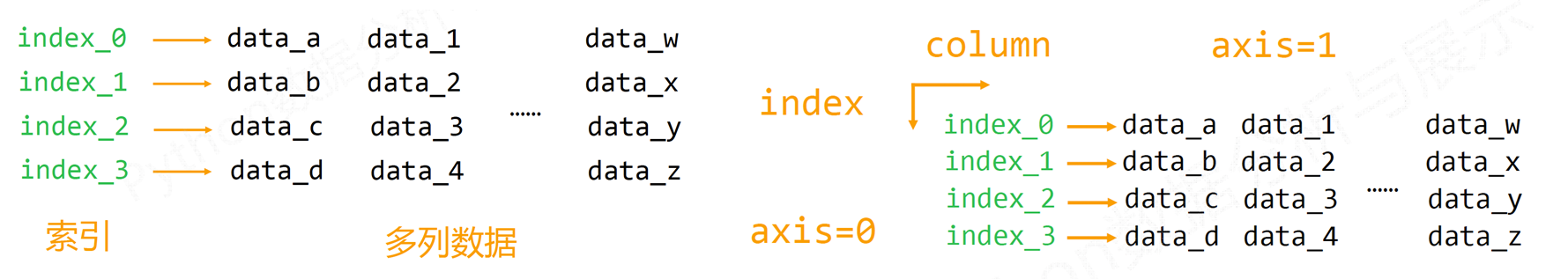### pandas的DataFrame类型创建

DataFrame类型可以由如下类型创建：

• 二维ndarray对象
• 由一维ndarray、列表、字典、元组或Series构成的字典
• Series类型
• 其他的DataFrame类型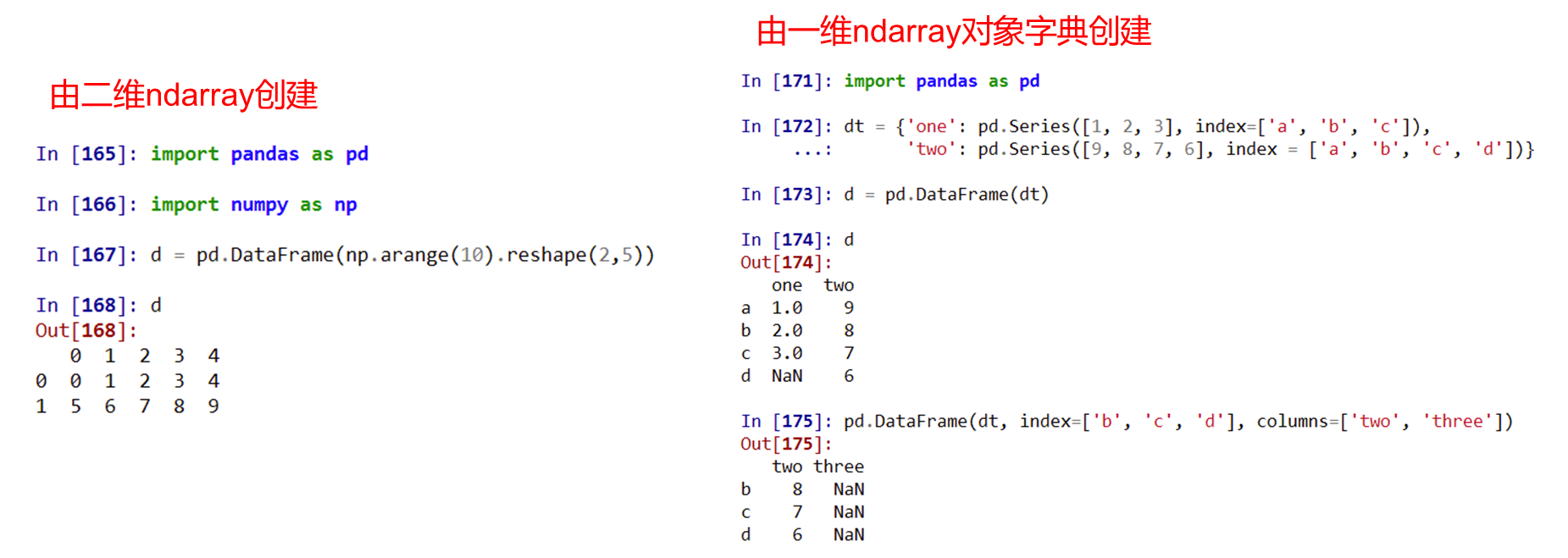### Pandas的Dataframe类型的基本操作

#### pandas索引操作

##### pandas重新索引

reindex()能够改变或重排Series和DataFrame索引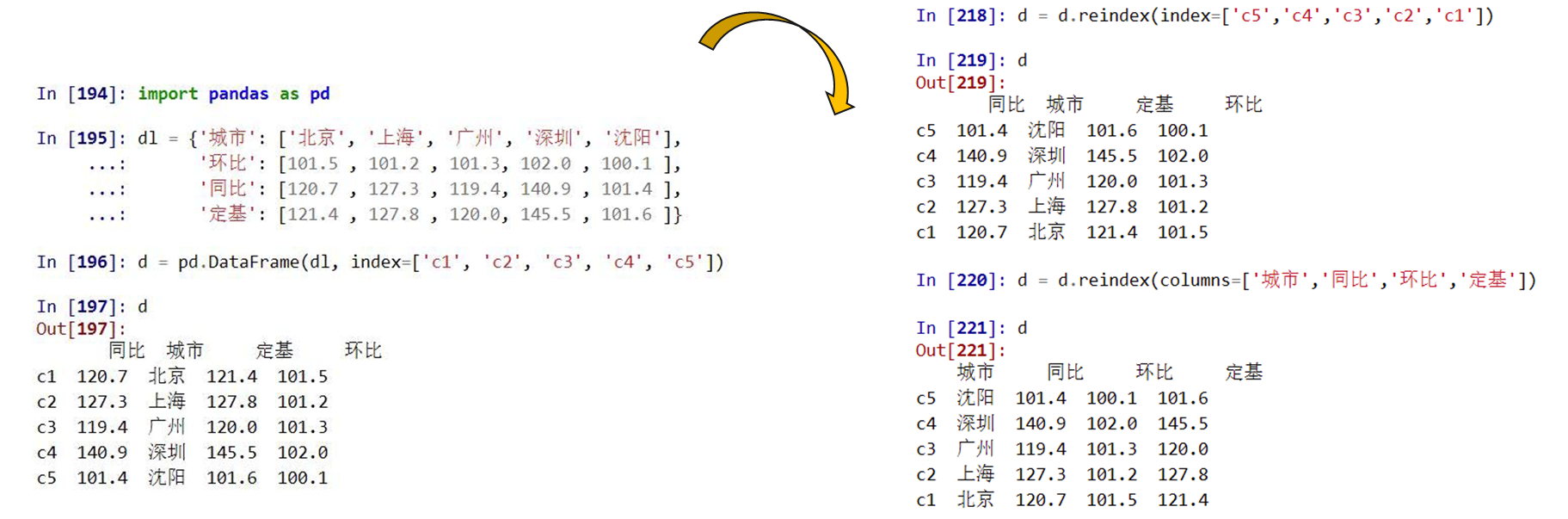reindex(index=None, columns=None, …)的参数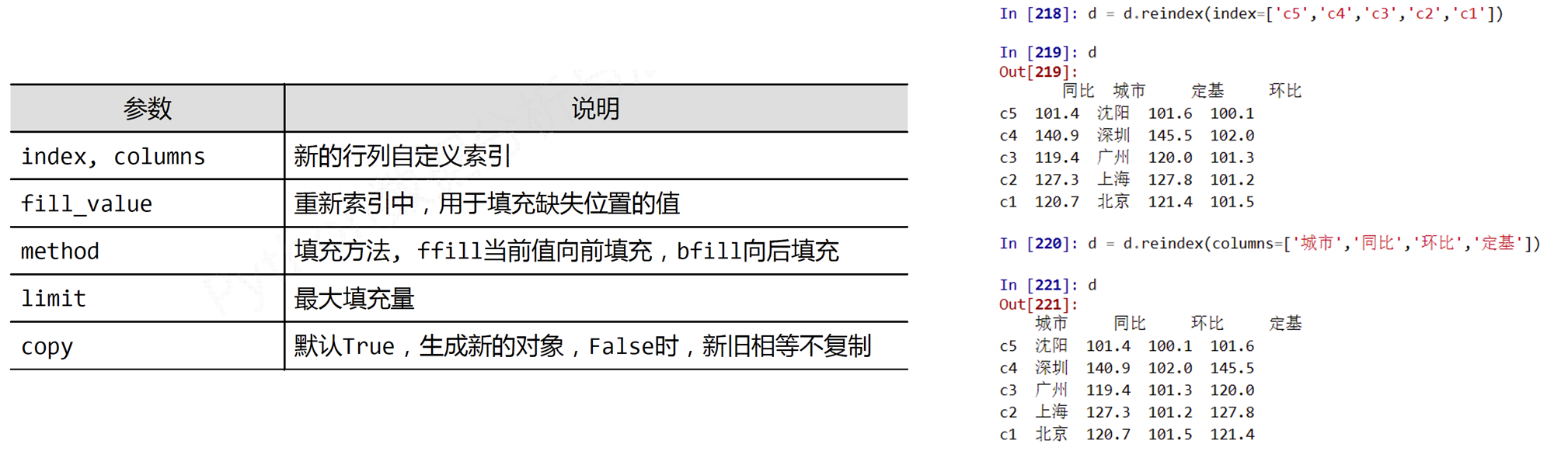pandas删除索引

drop()能够删除Series和DataFrame指定行或列索引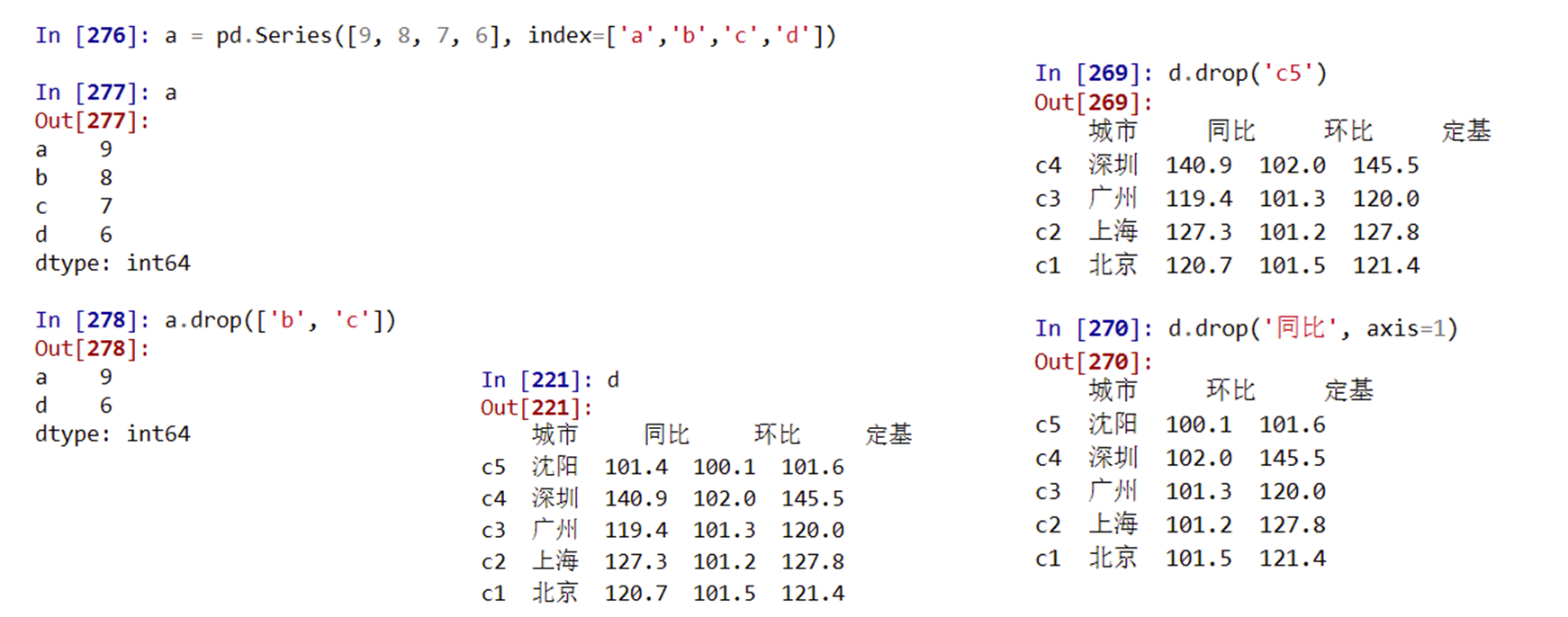#### pandas数据运算

• 算术运算根据行列索引，补齐后运算，运算默认产生浮点数
• 补齐时缺项填充NaN (空值)
• 二维和一维、一维和零维间为广播运算
• 采用+ ‐ * /符号进行的二元运算产生新的对象##### 算术运算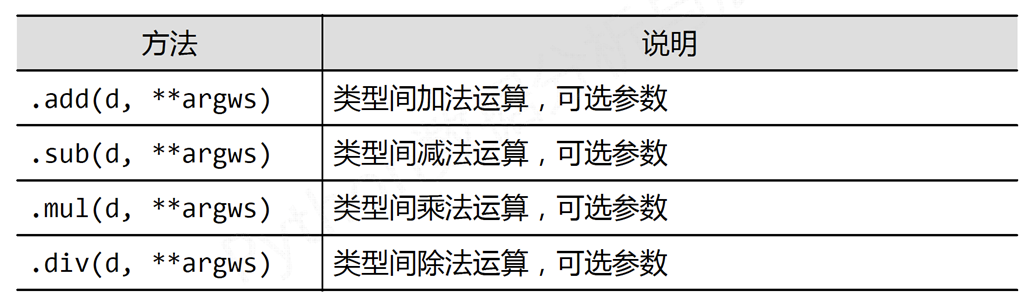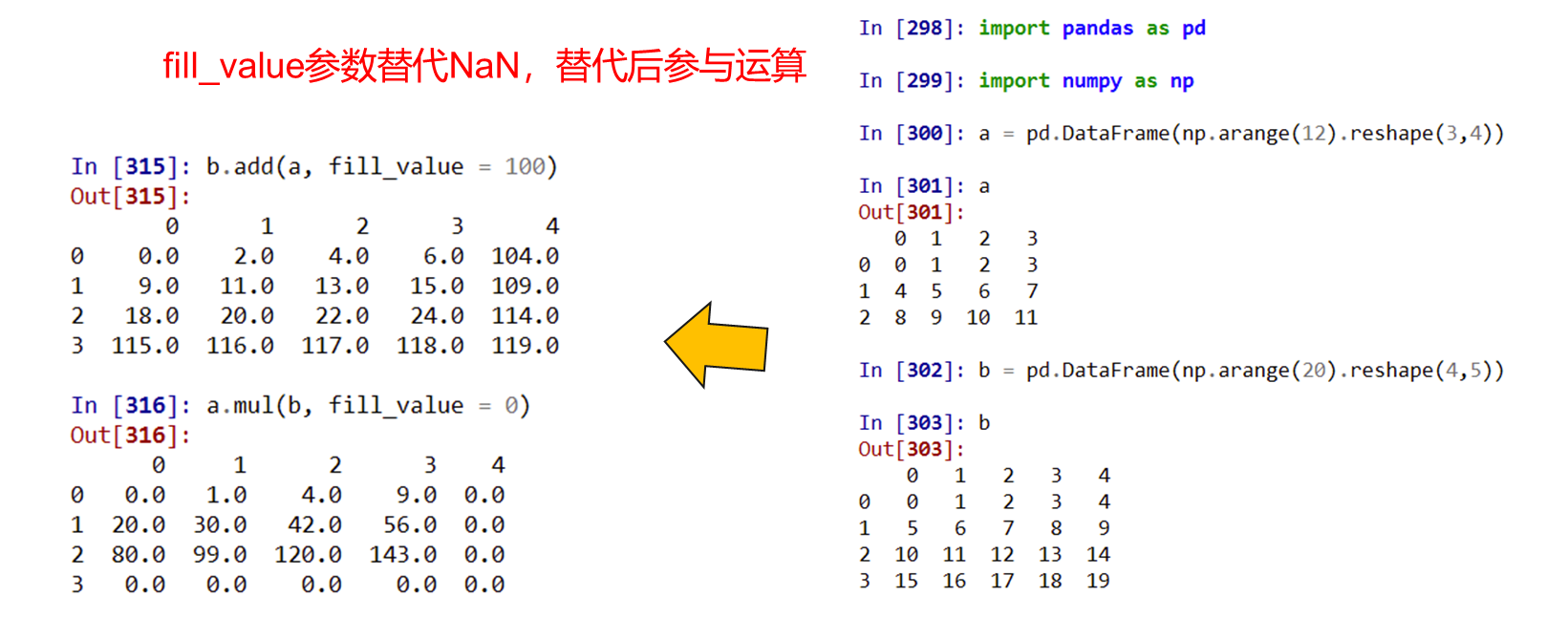• 不同维度间为广播运算，一维Series默认在轴1参与运算
• 使用运算方法可以令一维Series参与轴0运算

## Pandas数据分析

### Pandas导入与导出数据

#### 导入数据

pd.DataFrame(dict)：从字典对象导入数据，Key是列名，Value是数据

#### 导出数据

df.to_csv(filename)：导出数据到CSV文件

df.to_excel(filename)：导出数据到Excel文件

df.to_sql(table_name, connection_object)：导出数据到SQL表

df.to_json(filename)：以Json格式导出数据到文本文件

### Pandas查看、检查数据

df.tail(n)：查看DataFrame对象的最后n行

df.shape()：查看行数和列数

df.info()：查看索引、数据类型和内存信息

df.describe()：查看数值型列的汇总统计

s.value_counts(dropna=False)：查看Series对象的唯一值和计数

df.apply(pd.Series.value_counts)：查看DataFrame对象中每一列的唯一值和计数

### Pandas数据选取

df[col]：根据列名，并以Series的形式返回列

df[[col1, col2]]：以DataFrame形式返回多列

s.iloc：按位置选取数据

s.loc[‘index_one’]：按索引选取数据

df.iloc[0,:]：返回第一行

df.iloc[0,0]：返回第一列的第一个元素

### pandas数据清理

df.columns = [‘a’,’b’,’c’]：重命名列名

pd.isnull()：检查DataFrame对象中的空值，并返回一个Boolean数组

pd.notnull()：检查DataFrame对象中的非空值，并返回一个Boolean数组

df.dropna()：删除所有包含空值的行

df.dropna(axis=1)：删除所有包含空值的列

df.dropna(axis=1,thresh=n)：删除所有小于n个非空值的行

df.fillna(x)：用x替换DataFrame对象中所有的空值

s.astype(float)：将Series中的数据类型更改为float类型

s.replace(1,’one’)：用‘one’代替所有等于1的值

s.replace([1,3],[‘one’,’three’])：用’one’代替1，用’three’代替3

df.rename(columns=lambda x: x + 1)：批量更改列名

df.rename(columns={‘old_name’: ‘new_ name’})：选择性更改列名

df.set_index(‘column_one’)：更改索引列

df.rename(index=lambda x: x + 1)：批量重命名索引

### Pandas数据处理

df.columns = [‘a’,’b’,’c’]：重命名列名

pd.isnull()：检查DataFrame对象中的空值，并返回一个Boolean数组

pd.notnull()：检查DataFrame对象中的非空值，并返回一个Boolean数组

df.dropna()：删除所有包含空值的行

df.dropna(axis=1)：删除所有包含空值的列

df.dropna(axis=1,thresh=n)：删除所有小于n个非空值的行

df.fillna(x)：用x替换DataFrame对象中所有的空值

s.astype(float)：将Series中的数据类型更改为float类型

s.replace(1,’one’)：用‘one’代替所有等于1的值

s.replace([1,3],[‘one’,’three’])：用’one’代替1，用’three’代替3

df.rename(columns=lambda x: x + 1)：批量更改列名

df.rename(columns={‘old_name’: ‘new_ name’})：选择性更改列名

df.set_index(‘column_one’)：更改索引列

df.rename(index=lambda x: x + 1)：批量重命名索引

df[df[col] > 0.5]：选择col列的值大于0.5的行

df.sort_values(col1)：按照列col1排序数据，默认升序排列

df.sort_values(col2, ascending=False)：按照列col1降序排列数据

df.sort_values([col1,col2], ascending=[True,False])：先按列col1升序排列，后按col2降序排列数据

df.groupby(col)：返回一个按列col进行分组的Groupby对象

df.groupby([col1,col2])：返回一个按多列进行分组的Groupby对象

df.groupby(col1)[col2]：返回按列col1进行分组后，列col2的均值

df.pivot_table(index=col1, values=[col2,col3], aggfunc=max)：创建一个按列col1进行分组，并计算col2和col3的最大值的数据透视表

df.groupby(col1).agg(np.mean)：返回按列col1分组的所有列的均值

data.apply(np.mean)：对DataFrame中的每一列应用函数np.mean

data.apply(np.max,axis=1)：对DataFrame中的每一行应用函数np.max

#### Pandas数据合并

df1.append(df2)：将df2中的行添加到df1的尾部

df.concat([df1, df2],axis=1)：将df2中的列添加到df1的尾部

df1.join(df2,on=col1,how=’inner’)：对df1的列和df2的列执行SQL形式的join

#### Pandas数据统计

df.describe()：查看数据值列的汇总统计

df.mean()：返回所有列的均值

df.corr()：返回列与列之间的相关系数

df.count()：返回每一列中的非空值的个数

df.max()：返回每一列的最大值

df.min()：返回每一列的最小值

df.median()：返回每一列的中位数

df.std()：返回每一列的标准差

目录